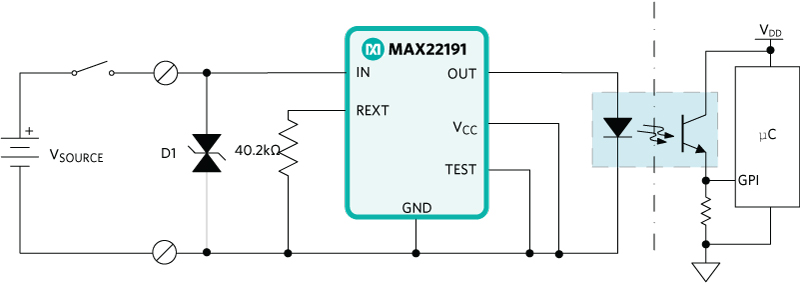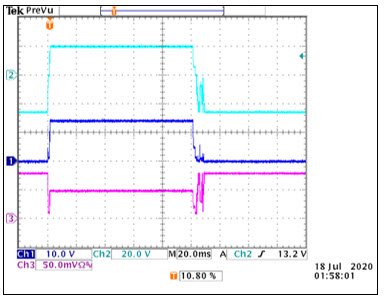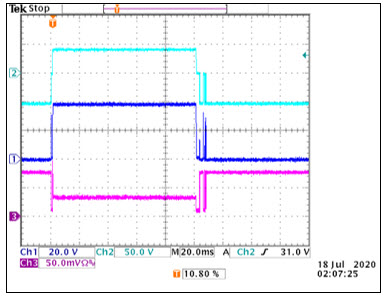# Industrial Digital Inputs with the MAX22191

### 要約

A digital input (DI) is a circuit designed to receive a binary signal transmitted from an industrial sensor and translate that input into a reliable logic signal for a programmable logic controller (PLC) or industrial controller. Common examples of industrial binary signals are pushbuttons and/or temperature or proximity threshold indicators. The MAX22191 parasitically powered DI circuit can monitor Type 1 and Type 3 sink and source binary input signals for PLC and industrial circuits. This application note shows examples of current sink, current source, high voltage, and AC detection digital input circuits using the MAX22191.

#### Introduction

A DI is a circuit designed to receive a binary signal transmitted from an industrial sensor and translate that input into a reliable logic signal for a PLC or industrial controller. Common examples of industrial binary signals are pushbuttons and/or temperature or proximity threshold indicators. The MAX22191 parasitically powered DI circuit can monitor Type 1 and Type 3 sink and source binary input signals for PLC and industrial circuits.

#### Types of Digital Inputs

The IEC 61131-2 standard governs the input threshold and current requirements for sinking DI circuits in industrial applications and defines three types of DI circuits for industrial applications:

• Type 1: Electromechanical circuits
• Type 2: Discrete, high current, semiconductor circuits
• Type 3: Integrated (low power) semiconductor circuits

The differences between the DI types are primarily voltage thresholds and current limits. Additionally, DI circuits are typically used in one of two configurations: positive logic (sinking inputs and sourcing outputs), negative logic (sourcing inputs and sinking outputs). The IEC 61131-2 thresholds for 24V and 48V (DC and AC) sinking inputs are shown in Table 1.

Table 1. IEC 61131-2 Type 1-3 Definitions

 Type of Limit Type 1 Limits Type 2 Limits Type 3 Limits Off Region Transition On Region Off Region Transition On Region Off Region Transition On Region VL (V) IL (mA) VL (V) IL (mA) VL (V) IL (mA) VL (V) IL (mA) VL (V) IL (mA) VL (V) IL (mA) VL (V) IL (mA) VL (V) IL (mA) VL (V) IL (mA) Max 15/5 15 15 15 30 15 11/5 30 11 30 30 30 11/5 15 11 15 30 15 Min -3 ND 5 05 15 2 -2 ND 5 2 11 6 -3 ND 5 1.5 11 2

As sensor density increases on factory floors, digital input circuits with smaller packages and lower power dissipation are required. As seen in the table, Type 2 DI circuits require a larger amount of power and are, in turn, naturally phasing out of modern industrial equipment.

The MAX22191 was designed to support Type 1 (DC and AC) and Type 3 (DC) inputs.

#### 24V DC Sinking Digital Input

A common DI circuit is the positive logic circuit, also known as a sinking input. In a sinking input configuration, the DI circuit sinks current from the source when the source voltage is high. Figure 1 shows the MAX22191 in a parasitically powered sinking input configuration.Figure 1. MAX22191 current sinking DI circuit.

When the input voltage (at the IN pin) exceeds the on-state voltage threshold (10V, max for the MAX22191), the MAX22191 detects an ON-state. The output is then enabled and sources 2.3mA (typ) of current to the load. When the input voltage falls below the IN voltage lower threshold (7V, min) the output current drops to 0mA, signaling an OFF-state. Using the circuit shown in Figure 1 with VSOURCE = 24V and a relay to pulse the input of the circuit, the IN pin voltage and OUT current of the MAX22191 are shown in Figure 2.Figure 2. MAX22191 IN and OUT signal in current sinking circuit, CH1 = IN, CH3 = IOUT x 10O.

Note that the output source current is represented by the CH3 waveform. Calculate the output (OUT) source current by dividing the CH3 waveform by 10O.

Note that the D1 TVS diode is in place to protect the MAX22191 during high voltage transient events. When selecting a D1 diode, ensure that its clamping voltage is below the absolute maximum rating of the input pin (+60V for the MAX22191 IN pin).

#### 24V DC Sourcing Digital Input

A negative logic DI circuit features a current-sourcing input and current-sinking output. This type of configuration is a current-sourcing DI circuit. Note that special care should be used when using the current-sourcing DI circuit, as short-circuit conditions result in an output "on-state."

Figure 3 shows the MAX22191 in a parasitically powered current source configuration.Figure 3. MAX22191 current sourcing circuit.

In this configuration, the MAX22191 IN pin connects to the 24V power supply, and the sensor or switch connects the GND/return terminal to the GND terminal of the signal source. This circuit is in the ON-state only when the switch is closed and current can flow to the return terminal of the source. The resulting waveform, referenced to the return terminal, is similar to the waveform in Figure 2.

#### Using the MAX22191 with a Status/Indicator LED

The MAX22191 can parasitically power itself, an optical isolator, and an external LED for status information, making it the lower power digital isolation solution available. Figure 4 shows a sample current sink configuration with the external LED.Figure 4. MAX22191 current sinking circuit with status/indicator LED.

When the input voltage (VIN) exceeds the input-high threshold, the output sources 2.3mA (typ) current and offers a voltage compliance that is enough to drive both an external status LED and an optical isolator. When the input voltage drops below the input-low threshold, the output current is cut off and the LED is thus turned off, and the optical isolator is off.

#### High Voltage and AC Signal Detection

Some applications require digital inputs suitable for sinking, sourcing, and large AC input signal voltages. The addition of an external diode bridge and a few resistors allow the MAX22191 to be used in systems with these requirements. Figure 5 shows the basic MAX22191 circuit for high-voltage bidirectional signal detection.Figure 5. Bidirectional high-voltage detection circuit for MAX22191.

Two 1.5kO MELF resistors and two TVS diodes D1 and D2 are used to protect the circuit against transient high-voltage/current events like surge (IEC 61000-4-5), electrical fast transient (EFT, IEC 61000-4-5), and electrostatic discharge (ESD, IEC 61000-4-2). Ensure that the D1 and D2 clamping voltages are below the maximum rating for the DI input pin.

The D3 diode bridge is used to rectify the incoming signal to 0V = V2 = VSOURCE. These added diodes also increase the sink/source current of the overall DI circuit and slightly increase the overall power dissipation of the circuit. Using the circuit in Figure 4 with a ±24V VSOURCE input, the waveforms in Figure 6 show the IN input voltage and OUT output source current during switching. Figure 7, showing the same waveforms, uses a ±48V VSOURCE voltage.Figure 6. MAX22191 bidirectional circuit signals with ±24V input signal, CH2 = Terminal input, CH1 = IN of MAX22191, CH3 = IOUT x 10O.Figure 7. MAX22191 bidirectional circuit signals with ±48V input signal.
CH2 = Terminal input, CH1 = IN of MAX22191, CH3 = IOUT x 10O

Resistors R1 and R2 in Figure 5 protect the MAX22191 in high-voltage applications and can be used to set the on-state trigger voltage if higher than 10V. Calculate the resistor values for a selected threshold (VTH) using the following steps:

1. Calculate the V2 voltage when VSOURCE = VTH as V2 = VSOURCE - [VDIODE + (1.5kO x IIN)].
2. Calculate V1 = V2 - (1.5kO x IIN).
3. Select R2 between 100O and 1kO.
4. Calculate R1 = R2 x (V1/10 - 1).

Set IIN = 3.8mA for bidirectional input calculations.

For example, using a ±110V bidirectional input signal, let us assume that the trigger threshold has been set at +60V. Calculate the resistor divider values as follows:

1. V2 = 60V - (0.7V + 5.7V) = 53.6V
2. V1 = 53.6V - 5.7V = 47.9V
3. R2 = 1kO
4. R1 = 1kO x (47.9/10 - 1) = 3.79kO

Select the resistor sizes with appropriate power dissipation ratings for normal operation.

Although shown for a bidirectional input signal in Figure 5, a resistor divider can also be suitable for unidirectional input signal circuits. Calculations and power considerations for the unidirectional circuit are similar to the ones shown here, but the input current should be set at 2.4mA (IIN = 2.4mA).